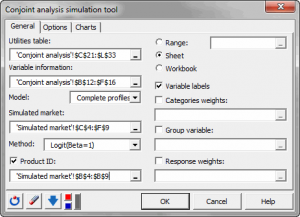# Simulation for conjoint analysis

Once a conjoint analysis has been performed, use the results to simulate the market share of a new product, in Excel using the XLSTAT software.## Principle of simulation for conjoint analysis

Conjoint analysis is a comprehensive method for the analysis of new products in a competitive environment.

Once the analysis has been performed, the major advantage of conjoint analysis is its ability to perform market simulations using the obtained utilities. The products included in the market do not have to be part of the tested products.

Outputs from conjoint analysis include utilities which can be partial (associated to each individual in full profile conjoint analysis) or aggregate (associated to all the individuals in CBC). These utilities allow computing a global utility associated to any product that you want to include in your simulated market.

Four estimation methods are proposed in XLSTAT-Conjoint: first choice, logit, Bradley-Terry-Luce and randomized first choice.

## Simulation for conjoint analysis

The obtained market shares can then be analyzed to assess the possible introduction of a new product on the market. The results of these simulations are nevertheless dependent on the knowledge of the real market and the fact that all important factors associated with each product in the conjoint analysis have been taken into account.

XLSTAT-Conjoint analysis software can also add weights to the categories of the factors or to the individuals.

XLSTAT-Conjoint can also take into account groups of individuals when a group variable (segmentation) is available. It can be obtained, for example, with the segmentation tool associated with the conjoint analysis.

## Data type for simulation

XLSTAT-Conjoint proposes two models for conjoint analysis. In a full profile analysis, a constant is associated to the utilities and there are as many utilities as individuals in the study. You have to select all the utilities and their constant. In the case of CBC, there is no constant and you have to use the utilities without the labels associated to the name of the categories.

## Simulation algorithms

Simulation methods XLSTAT-Conjoint offers four methods for simulation of market share. The first step consists of calculating the global utility associated with each new product. Thus, for a CBC analysis for analyzing men's shoes with three factors: the price (50 dollars, 100 dollars, 150 dollars), their finishing (canvas, leather, suede) and the color (brown, black). We have a table with 8 partial utilities rows and one column. We want to simulate a market with a black leather shoe with price equal USD 100. The utility of this product is: UP1 = Uprice-100 + UF-Leather + UC-Black. We calculate the utility for each product in the market and we seek the probability of choosing this product using different estimation methods:

• First choice: it is the most basic; you select the product with maximum utility with a probability of 1.
• Logit: this method is based on the exponential function to find the probability, it is more accurate than the method first choice and it is generally preferred. It has the disadvantage of the IIA assumption (assumption of independence of irrelevant alternatives). It is calculated for the product P1: PP1 = eUP1β / Σi eUPiβ with beta = 1 or 2.
• Bradley-Terry-Luce is a method close to the logit method without using the exponential function. It always involves the assumption of IIA and demands positive utilities (if beta = 1). It is calculated for the product P1: PP1 = UP1β / Σi UPiββ with beta = 1 or 2.
• Randomized first choice: it is a method midway between logit and First Choice. It has the advantage of not assuming the IIA assumption and is based on a simple principle: it generates a large number of numbers from a Gumbel distribution and creates a new set of utilities using the initial utilities adding the numbers generated. For each set of utilities created, the first choice method is used to select one of the products. So we will accept slight variations around the calculated values of the utilities. This method is the most advanced but also more suited to the case of conjoint analysis.
• RFC-Bolse: In the case of profile-based conjoint analysis, the Randomized First Choice BOLSE (RFC-BOLSE) was introduced to overcome the problems of the RFC method. Indeed, RFC is based on a Gumbel law that do not fit the full profile method. This approach is based on the same principle as Randomized First Choice but it uses a different distribution function to generate the simulated numbers. The RFC model adds unique random error (variation) to the part-worths and computes market shares using the First Choice rule. The centered normal distribution is used with standard error equal to the standard error of the parameters of the regression model and a global error term associated to the entire model. For each set of utilities created, the first choice method is used to select one of the products. So we will accept slight variations around the calculated values of the utilities. This method is the most advanced but also more suited to the case of profile based conjoint analysis.Randomized first choice: it is a method midway between logit and First Choice. It has the advantage of not assuming the IIA assumption and is based on a simple principle: it generates a large number of numbers from a Gumbel distribution and creates a new set of utilities using the initial utilities adding the numbers generated. For each set of utilities created, the first choice method is used to select one of the products. So we will accept slight variations around the calculated values of the utilities. This method is the most advanced but also more suited to the case of conjoint analysis.

When more than one column of utilities (with a conjoint analysis with full profiles) are selected, XLSTAT-Conjoint uses the mean of the probabilities.### analyze your data with xlstat

14-day free trial

Included in

Related features